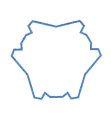Practice the questions of McGraw Hill Math Grade 8 Answer Key PDF Lesson 21.2 Polygons to secure good marks & knowledge in the exams.

Exercises

IDENTIFY

Indicate whether the figure is a polygon. Write “Polygon” or “Not a Polygon”.

Question 1.Polygon,

Explanation:
The given shape is a polygon as it is a closed 2-dimensional figure made up of line segments and it has more than 3 sides.

Question 2.Polygon,

Explanation:
The given shape is a polygon as it is a closed 2-dimensional figure made up of line segments and it has more than 3 sides.

Question 3.Polygon,

Explanation:
The given shape is a polygon as it is a closed 2-dimensional figure made up of line segments and it has more than 3 sides.

Question 4.Polygon,

Explanation:
The given shape is a polygon as it is a closed 2-dimensional figure made up of line segments and it has more than 3 sides.

Question 5.Not a Polygon,

Explanation:
The given shape is a not polygon as it is a closed 2-dimensional figure made up of not only line segments but also with curves.

Question 6.Polygon,

Explanation:
The given shape is a polygon as it is a closed 2-dimensional figure made up of line segments and it has more than 3 sides.

Question 7.Polygon,

Explanation:
The given shape is a polygon as it is a closed 2-dimensional figure made up of line segments and it has more than 3 sides.

Question 8.Polygon,

Explanation:
The given shape is a polygon as it is a closed 2-dimensional figure made up of line segments and it has more than 3 sides.

Question 9.